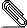# Re: [isabelle-dev] Poly/ML x86_64_32 available for testing

```On 1/22/2019 2:28 PM, Makarius wrote:
```
```On 22/01/2019 20:05, Fabian Immler wrote:
```
```These numbers look quite extreme:
The slowdown in polyml-5.7.1-8 is about a factor of 2-3 (and not 30 as
it seems to be the case with polyml-test-0a6ebca445fc).```
```
The following is isabelle/ab5a8a2519b0 afp/f49f0b0ead38 on lxcisa0:

ML_PLATFORM="x86-linux"
ML_OPTIONS="--minheap 2000 --maxheap 4000"
Finished HOL-ODE-Numerics (0:25:27 elapsed time, 1:07:49 cpu time,
factor 2.66)
Finished HOL-ODE-ARCH-COMP (0:18:01 elapsed time, 0:26:19 cpu time,
factor 1.46)
```
```
Can you point to some smaller parts of these sessions, where the effect
is visible? We can then use profiling to get an idea what actually
happens in x86_64_32.
```
It should be the by (tactic ...) invocations, which ultimately run generated code as an oracle (the one defined via @{computation_check} here: https://bitbucket.org/isa-afp/afp-devel/src/5d11846ac6abdad9c9dfee108d2772ac71c6179c/thys/Ordinary_Differential_Equations/Numerics/Example_Utilities.thy#lines-2449)
```
The term that is being evaluated should be printed when declaring
[[ode_numerics_trace]].
(But it takes a lot of time to get there...)

```
I would have assumed that it is the heavy use of int computations that makes the difference, and it should be precisely the kind that is tested in the attached Float_Test.thy.
```
```
On my Windows-Laptop and on lxcisa0, however, I see the expected slowdown of about a factor of 2, but not more...
```
Could the issue be related to specific machines?
```
(I am testing HOL-ODE-ARCH-COMP with polyml-test-0a6ebca445fc/x86_64_32-linux right now, but that does take some time...)
```
Fabian

```
```theory Float_Test
imports
"HOL-Library.Float"
"HOL-Library.Code_Target_Numeral"
begin

definition "logistic p r x =
normfloat (float_round_down p (float_round_down p (r * x)) * (float_plus_down
p 1 (-x)))"

primrec iter where
"iter p r x 0 = x"
| "iter p r x (Suc n) = iter p r (logistic p r x) n"

definition "logistic_chaos i = iter 30 (Float 15 (-4)) (Float 1 (-1))
(nat_of_integer i)"

ML \<open>val logistic_chaos = @{code logistic_chaos}\<close>
ML \<open>logistic_chaos 1000000\<close>

end```smime.p7s
Description: S/MIME Cryptographic Signature

```_______________________________________________
isabelle-dev mailing list
isabelle-...@in.tum.de
https://mailmanbroy.informatik.tu-muenchen.de/mailman/listinfo/isabelle-dev
```